# CELL

Definition:-

Spreadsheets can have multiple sheets, with each sheet having any number of rows or columns. A cell is a location at the intersection of a particular row and column, and may contain a data value.

• Cells are the boxes you see in the grid of an Excel worksheet.
• like this one Each cell is identified on a worksheet by its reference.
• The column letter and row number that intersect at the cell’s location. This cell is in column D and row 5, so it is cell D5.
• The column always comes first in a cell reference.
• Cell address and cell Reference is an alphanumeric value used to identified specific cell in spreadsheet .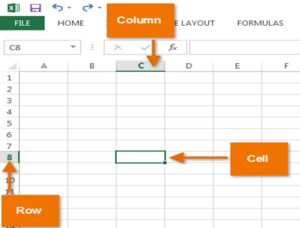# WORKSHEET

Definition:-

The term Worksheet used in Excel documents is a collection of cells organized in rows and columns. It is the working surface you interact with to enter data. Each worksheet contains 1048576 rows and 16384 columns and serves as a giant table that allows you to organize information.

• MS Excel is  also known as spreadsheet.
• A worksheet is a components of workbook.
• In the simple word’s the original meaning of a worksheet is a sheet of paper on which one performs work.
• A worksheet is a single spreadsheet of data.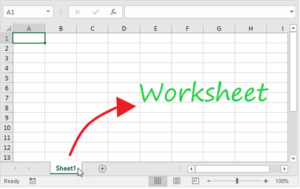# WORKBOOK

Definition:-

A workbook is an Excel file with one or more worksheets. A worksheet is a single spreadsheet of data. A workbook would be the entire binder, with everything in it. A worksheet would be like one section in that binder.

• In Excel ,a workbook is a collection of one or more spreadsheet, also called worksheet ,in single file.
• A workbook is a file that contains one or more worksheets to help us organize data.
• You can the rename the sheet names to more meaningful name i.e. Daily expenses, monthly budget, etc.
• For example a spreadsheet called “Sheet1” in an Excel workbook file called “Book1.” Or example also has the “Sheet2” and “Sheet3” sheet tabs, which are also part of the same workbook.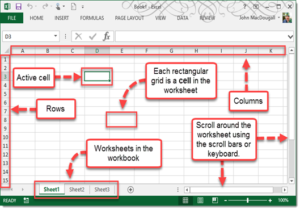# FORMULA

Definition:-

The formula tab allows us  to select from over 300 Excel functions. These functions are grouped into these categories: financial, logical, text, date & time, lookup and reference, math & trig, statistical, engineering, cube, information and compatibility.

A formula is an expression that operates on values in a range of cells or a cell. For example, =A1+A2+A3, which finds the sum of the range of values from cell A1 to cell A3.

These formulas return a result, even when it is an error. Excel formulas enable you to perform calculations such as addition, subtraction, multiplication, and division.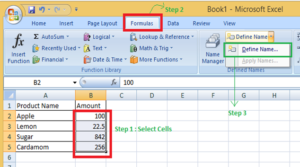# FUNCTION

Definition:-

Functions are predefined formulas that perform calculations by using specific values, called arguments, in a particular order, or structure. Functions can be used to perform simple or complex calculations. You can find all of Excel’s functions on the Formulas tab on the Ribbon: Excel function syntax.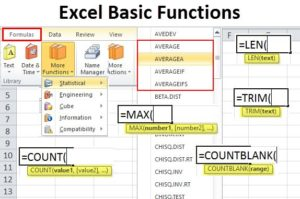The most frequently used functions in Excel are:

• AutoSum:

If you need to sum a column or row of numbers, let Excel do the math for you. Select a cell next to the numbers you want to sum, click AutoSum on the Home tab, press Enter, and you’re done.

Example: Autosum   [=sum(b2:c3)]

• MATCH function:

The MATCH function searches for a specified item in a range of cells, and then returns the relative position of that item in the range.

example, if the range A1:A3 contains the values 5, 25, and 38, then the formula =MATCH(25,A1:A3,0) returns the number 2, because 25 is the second item in the range.

-By Arti Rathia

error: Content is protected !!